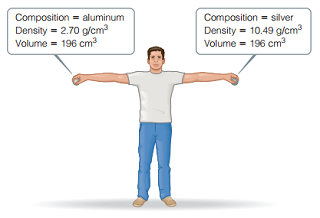# Problem: Consider the two spheres shown here , one made of silver and the other of aluminum. The spheres are dropped from a height of 2.1 m.What is the kinetic energy of the aluminum sphere at the moment it hits the ground? (Assume that energy is conserved during the fall and that 100% of the sphere’s initial potential energy is converted to kinetic energy by the time impact occurs.)

🤓 Based on our data, we think this question is relevant for Professor Van Dorn's class at ARIZONA.

###### FREE Expert Solution

We are being asked to calculate the kinetic energy of an aluminum sphere at the moment it hits the ground.

The aluminum sphere has an energy E which is the total of its potential and kinetic energy.

Before the aluminum sphere is dropped, all of its energy is potential energy. Potential energy is given by the formula:

where:

m is the mass

g is the Earth's gravity (9.81 m/s2)

h is the height###### Problem Details

Consider the two spheres shown here , one made of silver and the other of aluminum. The spheres are dropped from a height of 2.1 m.What is the kinetic energy of the aluminum sphere at the moment it hits the ground? (Assume that energy is conserved during the fall and that 100% of the sphere’s initial potential energy is converted to kinetic energy by the time impact occurs.)